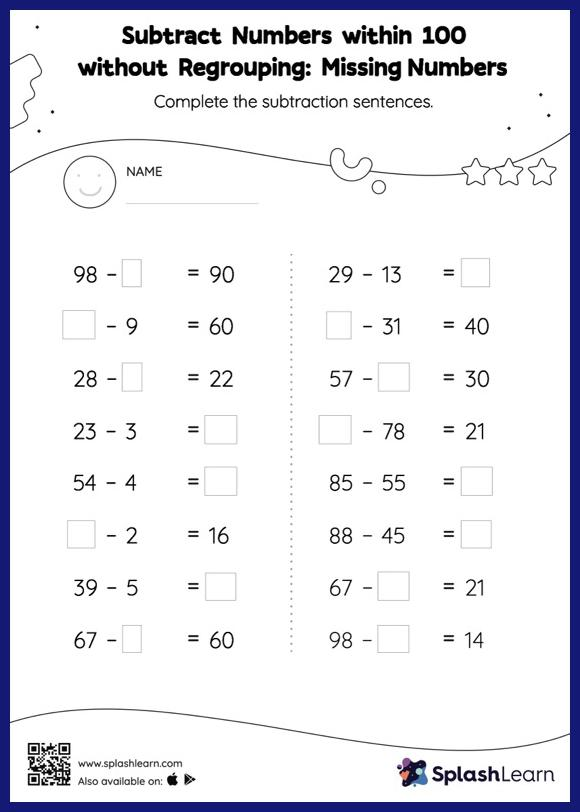# Subtract Numbers within 100 without Regrouping: Missing Numbers Worksheet

Home > Subtract Numbers within 100 without Regrouping: Missing NumbersCan your young mathematician subtract numbers within 100 without regrouping? That's exactly what they do here. To find the missing number on this worksheet, students can utilize the count back strategy or the connection between addition and subtraction. In subtract numbers within 100 without regrouping worksheet, they do not need to regroup numbers to reach the solution. This worksheet is about practicing with the horizontal format in which numbers are written side by side. To develop flexibility with numbers and operations, students need to have enough practice in this format and not just rely on the vertical/column method.